# ISEE Middle Level Quantitative : How to find perimeter

## Example Questions

← Previous 1 3 4 5 6 7 8 9 10

### Example Question #4 : Plane Geometry

What is the perimeter of a square with area 196 square inches?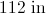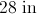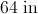It cannot be determined from the information given.Explanation:

A square with area 196 square inches has sidelength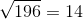inches, and therefore has perimeter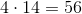inches

### Example Question #5 : Quadrilaterals

If a square has sides measuring, what is the perimeter of the square, in simplest form?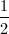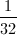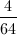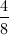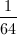Explanation:

To find the perimeter of a square, you must add together all the sides. In this case, we are addingfour times.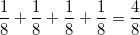Since all of the denominators are the same, there is no need to find a commond denominator, so we add together the numerators.  This gives us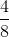.

Since both the numerator and denomator are divisible by four, we must simplify this fraction.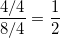The perimeter of the square is.

### Example Question #1 : How To Find Perimeter

The sum of the lengths of three sides of a square is one foot. Give the perimeter of the square in inches.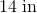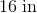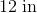It is impossible to determine the perimeter from the information given.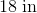Explanation:

A square has four sides of the same length.

One foot is equal to twelve inches; since the sum of the lengths of three of the congruent sides is twelve inches, each side measures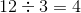inches.

The perimeter is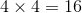inches.

### Example Question #2 : How To Find Perimeter

A square has perimeter one yard. Which is the greater quantity?

(A) The length of one side of the square

(B) 8 inches

(B) is greater

(A) and (B) are equal

(A) is greater

It is impossible to determine which is greater from the information given

(A) is greater

Explanation:

One yard is equal to 36 inches. A square has four sides of equal length, so one side of the square has length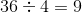inches.

Since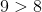, (A) is greater.

### Example Question #3 : How To Find Perimeter

A square has perimeter five meters. Which is the greater quantity?

(A) 1,250 millimeters

(B) The length of one side of the square

It is impossible to determine which is greater from the information given

(B) is greater

(A) and (B) are equal

(A) is greater

(A) and (B) are equal

Explanation:

One meter is equal to 1,000 millimeters, so the square has perimeter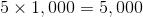millimeters.

A square has four sides of equal length, so one side of the square has length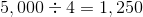millimeters.

The quantities are equal.

### Example Question #4 : How To Find Perimeter

A square lot has perimeter one mile. Which is the greater quantity?

(A) 1,320 feet

(B) The length of one side of the square

It is impossible to tell which is greater from the information given

(B) is greater

(A) is greater

(A) and (B) are equal

(A) and (B) are equal

Explanation:

One mile is equal to 5,280 feet. A square has four sides of equal length, so one side of the square has length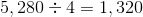feet.

The quantities are equal.

### Example Question #5 : How To Find Perimeter

The sum of the lengths of three sides of a square is 9,000 centimeters. Give its perimeter in meters.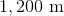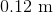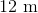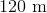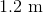Explanation:

100 centimeters are equal to one meter, so 9,000 centimeters are equal to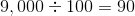meters.

A square has four sides of the same length. Since the sum of the lengths of three of the congruent sides is 9,000 centimeters, or 90 meters, each side measures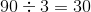meters.

The perimeter is four times this, which is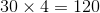meters.

### Example Question #5 : How To Find Perimeter

A square has perimeter one meter. Which is the greater quantity?

(A) 250 centimeters

(B) The length of one side of the square

(A) and (B) are equal

It is impossible to tell which is greater from the information given

(B) is greater

(A) is greater

(A) is greater

Explanation:

One meter is equal to 100 centimeters.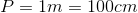A square has four sides of equal length, so we will need to divide by 4 to find the length of one side.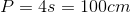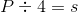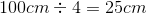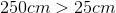, so (A) is greater

### Example Question #6 : How To Find Perimeter

Each side of a square is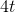units long. Which is the greater quantity?

(A)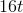(B) The perimeter of the square

(B) is greater

(A) and (B) are equal

(A) is greater

It is impossible to determine which is greater from the information given

(A) and (B) are equal

Explanation:

The perimeter of a square is four times its side length: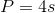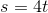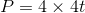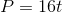Since the perimeter is equal to, (A) and (B) are equal.

### Example Question #8 : How To Find Perimeter

Hexagon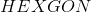and Square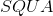both have perimeter 64. Which is the greater quantity?

(a)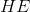(b)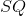(b) is the greater quantity

It is impossible to determine which is greater from the information given

(a) is the greater quantity

(a) and (b) are equal

The length of one side of the square - in particular, that of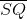- is equal to one fourth its perimeter, so it can be determined that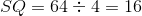. However, no relation among the sides of the hexagon is given - in particular, it is not given that the hexagon is regular - so the length of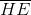cannot be determined. Insufficient information is given.